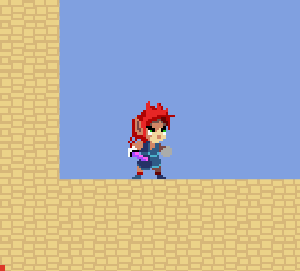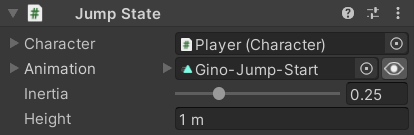﻿ Platformer Game Kit - Jump

# JumpThis state requires the character to be on the ground and launches them into the air with an amount of force calculated so that they will reach a specific `Height` at the top of their jump arc. The Player doesn't actually use this state directly, but all the other jump states Inherit from it to build upon its basic functionality.

# Fields

The field tooltips explain what they each do:

``````public class JumpState : CharacterState
{
[SerializeField]
private ClipTransition _Animation;
public ClipTransition Animation => _Animation;

[SerializeField, Range(0, 1)]
[Tooltip("Before the jump force is applied, your previous vertical velocity is multiplied by this value")]
private float _Inertia = 0.25f;
public float Inertia => _Inertia;

[SerializeField, Meters]
[Tooltip("The peak height of the jump arc")]
private float _Height = 3;
public float Height => _Height;
``````

The `OnValidate` ensures that the fields always have valid values:

``````#if UNITY_EDITOR
protected override void OnValidate()
{
base.OnValidate();
PlatformerUtilities.Clamp(ref _Inertia, 0, 1);
PlatformerUtilities.NotNegative(ref _Height);
}
#endif
``````

# Initialization

On startup, it sets the animation's End Event to return to the character's default state (the `Character.Idle` state):

``````    protected virtual void Awake()
{
_Animation.Events.OnEnd += Character.StateMachine.ForceSetDefaultState;
}
``````

# State Life Cycle

This state can only be entered while the character is on the ground:

``````    public override bool CanEnterState => Character.Body.IsGrounded;
``````

When entering this state, it calculates the velocity necessary to reach the desired `Height` (see Velocity Calculation), disables the Character Body so that it won't waste performance on any of its ground checks during the jump animation, and play the animation:

``````    public override void OnEnterState()
{
base.OnEnterState();

Character.Body.Velocity = CalculateJumpVelocity();
Character.Body.enabled = false;

Character.Animancer.Play(_Animation);
}
``````

During the jump animation, the character can move normally:

``````    public override float MovementSpeedMultiplier => 1;
``````

Once the End Event registered in `Awake` returns the character to their Idle state, it re-enables the Character Body so it can resume checking whether the character is on the ground or not:

``````    public override void OnExitState()
{
base.OnExitState();
Character.Body.enabled = true;
}
``````

# Velocity Calculation

Normally, the jump velocity calculation is based on the `_Inertia` and `_Height` fields of this class, but the method is `virtual` so that the Advanced Jump State can `override` it to use different values for wall jumps and air jumps:

``````    public virtual Vector2 CalculateJumpVelocity() => CalculateJumpVelocity(_Inertia, _Height);
``````

The calculation simply multiplies the character's vertical speed by the given `inertia` value then adds the speed necessary to reach the target `height`:

``````    public Vector2 CalculateJumpVelocity(float inertia, float height)
{
var velocity = Character.Body.Velocity;
velocity.y *= inertia;
velocity.y += CalculateJumpSpeed(height);
return velocity;
}
``````

The mathematical theory behind the calculation is a bit advanced and will not be explained here, but the resulting formula is actually a simple function of the target height and gravity:

``````    public float CalculateJumpSpeed(float height)
{
var gravity = Character.Body.Gravity.y;
AnimancerUtilities.Assert(gravity < 0, "Gravity is not negative");
return Mathf.Sqrt(-2 * gravity * height);
}
}
``````

That calculation requires gravity to be pointing downwards because the system doesn't currently support rotated characters or rotated gravity. Adding support for those things would be possible, but would require more modifications throughout the system than just this function.